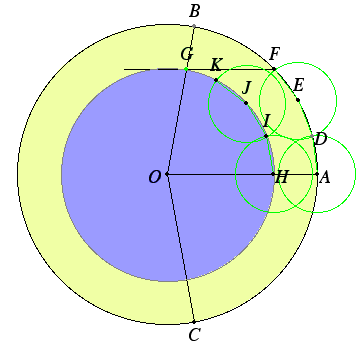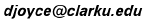### Trisect?

An idea for trisecting an angle. Take an arbritrary angle BOC. Let OA be the bisector of the angle. Draw a circle of arbitrary radius with O as center. Here the circle passes through A, B and C. Mark off three equal chords on that circle: AD, DE, and EF. Draw FG parallel to AO, and let G be that point where that line intersects OB. Draw the circle with center O and radius OG.. Now mark off three equal chords on that circle, each equal to the previous chords: HI, IJ, and JK. Then, for small angles, it looks like K = G. If K did equal G, then OI and OJ would trisect angle AOB, hence OJ would be one of the trisectors of BOC. Unfortunately, K is never equal to G. So this proposed method for trisecting angles doesn't work.It was proved in the 19th century that angles cannot be trisected with the Euclidean tools of straightedge and compass.